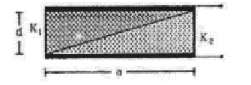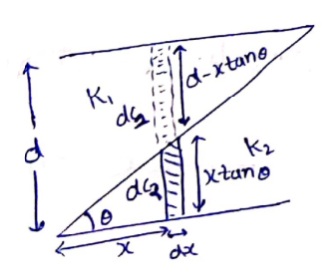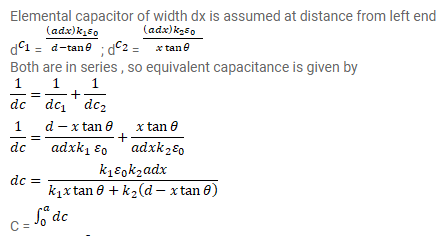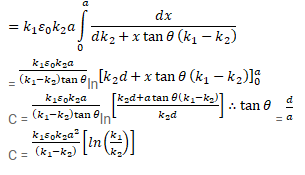# A capacitor is formed by two square metal-platesQuestion:

A capacitor is formed by two square metal-plates of edge a, separated by a distance $d$. Dielectrics of dielectric constants $K_{1}$ and $K_{2}$ are filled in the gap as shown in figure. Find the capacitance.Solution: